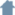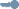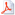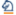# researchpublications

## international conferences

#### PLDI'08

“Discovering Properties about Arrays in Simple Programs”Array bound checking and array dependency analysis (for parallelization) have been widely studied. However, there are much less results about analyzing properties of array contents. In this paper, we propose a way of using abstract interpretation for discovering properties about array contents in some restricted cases: one-dimensional arrays, traversed by simple "for" loops. The basic idea, borrowed from [Gopan et al., 2005], consists in partitioning arrays into symbolic intervals (e.g., [1,i-1], [i,i], [i+1,n]), and in associating with each such interval I and each array A an abstract variable AI; the new idea is to consider relational abstract properties ψ(AI, BI, ...) about these abstract variables, and to interpret such a property pointwise on the interval I: &forall k &isin I, ψ(A[k], B[k],...). The abstract semantics of our simple programs according to these abstract properties has been defined and implemented in a prototype tool. The method is able, for instance, to discover that the result of an insertion sort is a sorted array, or that, in an array traversal guarded by a "sentinel", the index stays whithin the bounds.

#### VMCAI'07

“An Abstract Domain Extending Difference-Bound Matrices with Disequality Constraints”The property that two numerical variables always hold different values, at some point of a program, can be very useful, especially for analyzing aliases: if i ≠ j, then A[i] and A[j] are not aliased, and this knowledge is of great help for any other program analysis. Surprisingly, disequalities are seldom considered in abstract interpretation, most of the proposed numerical domains being restricted to convex sets. In this paper, we propose to combine simple ordering properties with disequalities. "Difference-bounds matrices" (or DBMs) is a domain proposed by David Dill, for expressing relations of the form "x - y ≤ c" or "c1 ≤ x ≤ c2". We define dDBMs ("disequalities DBM") as conjunctions of DBMs with simple disequalities of the form "x ≠ y" or "x ≠ 0". We give algorithms on dDBMs, for deciding the emptiness, computing a normal form, and performing the usual operations of an abstract domain. These algorithms have the same complexity (O(n3), where n is the number of variables) than those for classical DBMs, if the variables are considered to be valued in a dense set (R or Q). In the arithmetic case, the emptiness decision is NP-complete, and other operations run in O(n5).

• abstract domains, alias analysis, difference-bound matrices, disequalities, static analysis
• Lecture Notes in Computer Science, volume 4349• january 16, 2007, Plaza Hôtel, Nice, France• the references of the paper
• @InProceedings{PeronHalbwachsVMCAI07,
Author = {P\'eron, Mathias and Halbwachs, Nicolas},
Title = {An Abstract Domain Extending Difference-Bound Matrices with Disequality Constraints},
booktitle = {VMCAI'07: Eighth International Conference on Verification, Model Checking, and Abstract Interpretation},
pages = {268--282},
volume = {4349},
series = {Lecture Notes in Computer Science},
publisher = {Springer},
location = {Nice, France},
month = jan,
year = {2007},
}

• VMCAI 07, APRON, NBAC